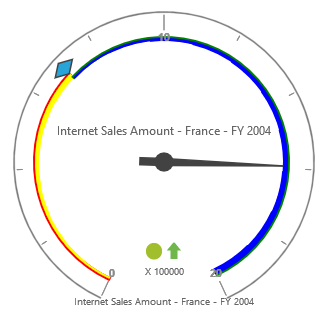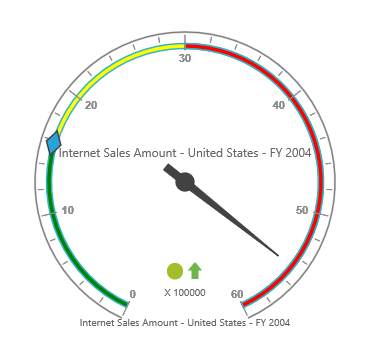# Ranges

The `RangeCollection` can be directly added to the scales option within the pivot gauge control.

• html
• ``````<ej:PivotGauge ID="PivotGauge1" runat="server">
<Scales>
<ej:CircularScales ShowRanges="true">
<RangeCollection>
<ej:CircularRanges DistanceFromScale="10"></ej:CircularRanges>
</RangeCollection>
</ej:CircularScales>
</Scales>
</ej:PivotGauge>``````

## Appearance customization

The appearance of the range can be customized through the following properties:

• StartValue: Defines the start position of the range.
• EndValue: Defines the end position of the range.
• StartWidth: Sets the width at starting position of the range.
• EndWidth: Sets the width at ending position of the range.
• BackgroundColor: Sets the background color of the range.
• Border: Sets the height and width of the border of the range.
• Placement: Sets the position of the range.
• DistanceFromScale: Sets the distance between the range and the scale.

Positioning the range can be set through the `Placement` or `DistanceFromScale` property.

NOTE

By default, the placement takes the value “near”, whereas other enumeration values available are “far” and “center”.

• html
• ``````<ej:PivotGauge ID="PivotGauge1" runat="server">
<Scales>
<ej:CircularScales ShowRanges="true">
<RangeCollection>
<ej:CircularRanges StartValue="20" EndValue="50" StartWidth="2" EndWidth="6" BackgroundColor="yellow" DistanceFromScale="20">
<Border Color="red" Width="2" />
</ej:CircularRanges>
<ej:CircularRanges StartValue="50" EndValue="100" StartWidth="2" EndWidth="7" BackgroundColor="blue" Placement="Near">
<Border Color="green" Width="2" />
</ej:CircularRanges>
</RangeCollection>
</ej:CircularScales>
</Scales>
</ej:PivotGauge>``````NOTE

When you set both the position properties - “DistanceFromScale” and “Placement” for a range, preference is given to the value that is set in the “DistanceFromScale” property.

## Multiple ranges

Multiple ranges can be added to the `RangeCollection` in the scales option within the pivot gauge control.

• html
• ``````<ej:PivotGauge ID="PivotGauge1" runat="server">
<Scales>
<ej:CircularScales ShowRanges="true">
<RangeCollection>
<ej:CircularRanges StartValue="0" EndValue="10" BackgroundColor="green" DistanceFromScale="-5"></ej:CircularRanges>
<ej:CircularRanges StartValue="10" EndValue="30" BackgroundColor="yellow" DistanceFromScale="-5"></ej:CircularRanges>
<ej:CircularRanges StartValue="30" EndValue="50" BackgroundColor="red" DistanceFromScale="-5"></ej:CircularRanges>
</RangeCollection>
</ej:CircularScales>
</Scales>
</ej:PivotGauge>``````